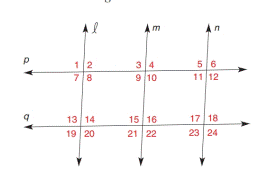Chapter 2.3, Problem 13E### Elementary Geometry for College St...

6th Edition
Daniel C. Alexander + 1 other
ISBN: 9781285195698

#### Solutions

Chapter
Section### Elementary Geometry for College St...

6th Edition
Daniel C. Alexander + 1 other
ISBN: 9781285195698
Textbook Problem
1 views

# In Exercise 7 to 16, name the lines (if any) that must be parallel under the given conditions.l ⊥ p and m ⊥ q

To determine

To find:

The parallel lines.

Explanation

Given:

The given statement is,

p and mq.

Approach:

If two coplanar lines are each perpendicular to a third line, then these lines are parallel.

Calculation:

The given statement is,

p and mq

### Still sussing out bartleby?

Check out a sample textbook solution.

See a sample solution

#### The Solution to Your Study Problems

Bartleby provides explanations to thousands of textbook problems written by our experts, many with advanced degrees!

Get Started

#### Calculate y'. 42. y=(x+)4x4+4

Single Variable Calculus: Early Transcendentals, Volume I

#### In Problems 35-42, simplify each complex fraction. 41.

Mathematical Applications for the Management, Life, and Social Sciences

#### In Exercises 7-28, perform the indicated operations and simplify each expression. 14. xexx+1+ex

Applied Calculus for the Managerial, Life, and Social Sciences: A Brief Approach

#### The center of 4x2 + 8x − 3y2 + 12y + 4 = 0 is (1, −2) (−1, 2) (2, −1) (−2, 1)

Study Guide for Stewart's Single Variable Calculus: Early Transcendentals, 8th